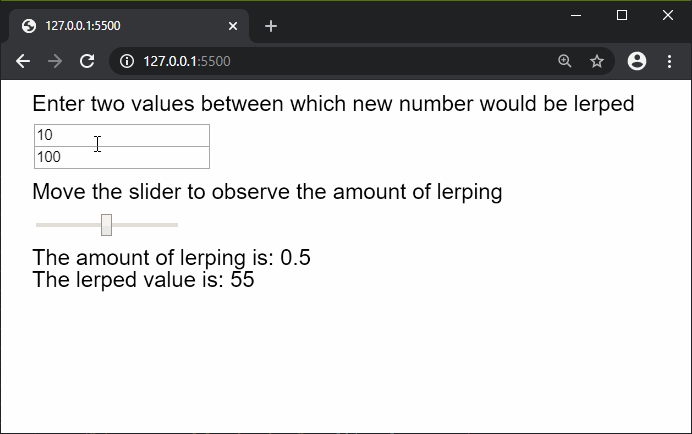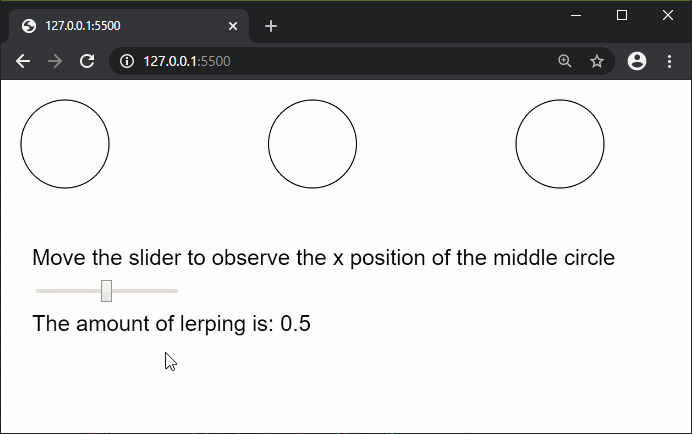GFG App
Open AppBrowser
Continue

The lerp() function is used to find a number between two numbers. The amt parameter can be used to specify the amount to interpolate between the two values. An amount nearer to 0.1 would mean that the final value is nearer to the first value, and nearer to 0.9 means that the value is nearer to the second value. If the value is less or more than these, then the final value would be calculated on the basis of the ratio of the two numbers.

It can be used for drawing dotted lines or creating motion along a path by finding all the intermediate points in a line.

Syntax:

`lerp( start, stop, amt )`

Parameters: This function accept three parameters as mentioned above and described below:

• start: It is a number which denotes the first value of the two numbers.
• stop: It is a number which denotes the second value of the two numbers..
• amt: It is a number which denotes the amount by which a number is to be interpolated between the two numbers.

Return Value: It returns a number with the lerped value.

Below examples illustrate the lerp() function in p5.js:

Example 1:

 `function` `setup() { ` `  ``createCanvas(600, 200); ` `  ``textSize(20); ` `  `  `  ``inputElemA = createInput(10); ` `  ``inputElemA.position(30, 40); ` `  `  `  ``inputElemB = createInput(100); ` `  ``inputElemB.position(30, 60); ` `  `  `  ``sliderElem = createSlider(0, 1, 0.5, 0.1); ` `  ``sliderElem.position(30, 120); ` `} ` `  `  `function` `draw() { ` `  ``clear(); ` `  ``text(``"Enter two values between which new "` `        ``+ ``"number would be lerped"``, 20, 20); ` `  ``text(``"Move the slider to observe the amount"` `        ``+ ``" of lerping"``, 20, 100); ` `  `  `  ``// Convert the string value to a number ` `  ``// value for lerping ` `  ``inputValA = Number(inputElemA.value()); ` `  ``inputValB = Number(inputElemB.value()); ` `  ``sliderVal = sliderElem.value(); ` `  `  `  ``text(``"The amount of lerping is: "` `        ``+ sliderVal, 20, 160); ` `         `  `  ``text(``"The lerped value is: "` `        ``+ lerp(inputValA, inputValB,  ` `        ``sliderVal), 20, 180); ` `} `

Output:Example 2:

 `function` `setup() { ` `  ``createCanvas(600, 300); ` `  ``textSize(20); ` ` `  `  ``sliderElem = createSlider(0, 1, 0.5, 0.1); ` `  ``sliderElem.position(30, 180); ` ` `  `  ``circleApos = 50; ` `  ``circleBpos = 500; ` `} ` ` `  `function` `draw() { ` `  ``clear(); ` `  ``text(``"Move the slider to observe the x position "` `          ``+ ``"of the middle circle"``, 20, 160); ` ` `  `  ``circle(circleApos, 50, 80); ` `  ``circle(circleBpos, 50, 80); ` ` `  `  ``sliderVal = sliderElem.value(); ` `  ``lerpedVal = lerp(circleApos, circleBpos, sliderVal); ` ` `  `  ``// Draw the circle at the lerped x coordinate ` `  ``circle(lerpedVal, 50, 80); ` ` `  `  ``text(``"The amount of lerping is: "` `+ sliderVal, 20, 220); ` `} `

Output:Online editor: https://editor.p5js.org/

Reference: https://p5js.org/reference/#/p5/lerp

My Personal Notes arrow_drop_up